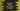# 6 ways in JavaScript to iterate over an array of objects## How to iterate over an array of objects in JavaScript:

There are different ways to loop through array in `JavaScript`. This post will show you 6 different ways with examples.

### Method 1: How to iterate over an object array with a `for` loop:

This is the traditional way to iterate over an array. The `for` loop uses a variable and it runs until a certain condition is `true`. We can run from `i = 0` to `i = array length - 1`. Since the array index starts at 0 and ends at array length - 1, we can access the array items with `i` as index position on each iteration.

At the end of each iteration, it will increment the value of `i` by 1.

The following program uses a `for` loop to iterate over an array of objects:

``````const arr = [{id: 1, name: 'Alex', age: 20}, {id: 2, name: 'Bob', age: 22}, {id: 3, name: 'Chandler', age: 21}];

for(let i = 0; i < arr.length; i++){
console.log(arr[i]);
}``````

It will run from `i = 0` to `i = arr.length - 1` and at the end of each iteration it increases the value of `i` by 1. If you run this program, it will print:

``````{ id: 1, name: 'Alex', age: 20 }
{ id: 2, name: 'Bob', age: 22 }
{ id: 3, name: 'Chandler', age: 21 }``````

### Method 2: By using a `while` loop:

The `while` loop checks a condition and executes the body of the loop until the condition is `true`. If we want to write the above example with a `while` loop, we need to initialize the value of `i` before the loop starts and increment its value at the end of each iteration.

``````const arr = [{ id: 1, name: 'Alex', age: 20 }, { id: 2, name: 'Bob', age: 22 }, { id: 3, name: 'Chandler', age: 21 }];

let i = 0;

while (i < arr.length) {
console.log(arr[i]);
i++;
};``````

It will print the same output.

### Method 3: By using a `do...while` loop:

The `do...while` loop is similar to a `while` loop. It runs the code in the `do` block before it checks the `while` condition.

``````const arr = [{ id: 1, name: 'Alex', age: 20 }, { id: 2, name: 'Bob', age: 22 }, { id: 3, name: 'Chandler', age: 21 }];

let i = 0;

do {
console.log(arr[i]);
i++;
}while(i < arr.length);``````

It will print the same result. But, the `do` block will run always as it checks the condition after the `do` block is executed. So, if you run the following program:

``````const arr = [];

let i = 0;

do {
console.log(arr[i]);
i++;
}while (i < arr.length);``````

It will print `undefined`. The `do` block will try to access the first element of `arr`, which is `undefined` as it is an empty array. We need to add an additional check to make this work:

``````const arr = [];

let i = -1;

do {
if(i >= 0){
console.log(arr[i]);
}
i++;
}while (i < arr.length);``````

### Method 4: Iterate over the array elements with a `forEach` method:

The `forEach` method executes a given function once for each element of the array. We can access the current array element and its index position in that function. The following example shows how to use the `forEach` method to iterate over an array of objects.

``````const arr = [{id: 1, name: 'Alex', age: 20}, {id: 2, name: 'Bob', age: 22}, {id: 3, name: 'Chandler', age: 21}];

arr.forEach((item, index)=> {
console.log(`Item at index \${index}: `, item);
});``````

If you run this program, it will print the following output:

``````Item at index 0:  { id: 1, name: 'Alex', age: 20 }
Item at index 1:  { id: 2, name: 'Bob', age: 22 }
Item at index 2:  { id: 3, name: 'Chandler', age: 21 }``````

### Method 5: By using a `for...of` loop:

The `for...of` loop provides direct access to the elements of an array. The syntax of this loop is:

``````for (item of iterable){

}``````

On each iteration, it will pick one `item` of the `iterable`. The following program uses a `for...of` loop to iterate over the objects of an array:

``````const arr = [{ id: 1, name: 'Alex', age: 20 }, { id: 2, name: 'Bob', age: 22 }, { id: 3, name: 'Chandler', age: 21 }];

for (let item of arr) {
console.log(item);
}``````

It will print:

``````{ id: 1, name: 'Alex', age: 20 }
{ id: 2, name: 'Bob', age: 22 }
{ id: 3, name: 'Chandler', age: 21 }``````

### Method 6: By using `for...in` loop:

The `for...in` loop is similar to the `for...of` loop. It is used to loop through an object. It provides the index of each element if we use `for...in` with an array.

``````const arr = [{ id: 1, name: 'Alex', age: 20 }, { id: 2, name: 'Bob', age: 22 }, { id: 3, name: 'Chandler', age: 21 }];

for (let i in arr) {
console.log(`arr[\${i}]: `, arr[i]);
}``````

It will print:

``````arr:  { id: 1, name: 'Alex', age: 20 }
arr:  { id: 2, name: 'Bob', age: 22 }
arr:  { id: 3, name: 'Chandler', age: 21 }``````